# Natural Convection Flow Past an Exponentially Accelerated Vertical Ramped Temperature Plate with Hall Effects and Heat Absorption

Natural Convection Flow Past an Exponentially Accelerated Vertical Ramped Temperature Plate with Hall Effects and Heat Absorption

G. S. SethR. Tripathi R. Sharma

Department of Applied Mathematics, Indian School of Mines, Dhanbad-826004, India

Corresponding Author Email:
gsseth_ism@yahoo.com
Page:
139-144
|
DOI:
http://doi.org/10.18280/ijht.330321
N/A
|
Accepted:
N/A
|
Published:
30 September 2015
| Citation

OPEN ACCESS

Abstract:

An investigation of unsteady MHD natural convection flow with Hall effects of an electrically conducting, viscous, incompressible and heat absorbing fluid past an exponentially accelerated vertical plate with ramped temperature through a porous medium in the presence of thermal diffusion is carried out. Exact solution for fluid velocity and fluid temperature is obtained in closed form by Laplace transform technique. The expressions for shear stress and rate of heat transfer at the plate are also derived. The numerical values of fluid velocity and fluid temperature are displayed graphically whereas those of shear stress and rate of heat transfer at the plate are presented in tabular form for various values of pertinent flow parameters.

Keywords:

Unsteady MHD natural convection, Hall current, Ramped temperature, Heat absorbing fluid, Exponentially accelerated plate.

1. Introduction

Effects of heat generation/absorption have significant contribution in the heat transfer characteristics of several physical problems of practical interest viz. convection in Earth’s mantle, post-accident heat removal, fire and combustion modeling, fluids undergoing exothermic and/or endothermic chemical reaction, development of metal waste from spent nuclear fuel, cooling of electronic components , applications in the field of nuclear energy etc. Therefore, it is appropriate to consider temperature dependent heat source and/or sink which may have strong influence on heat transfer characteristics of the fluid flow problems under consideration. Taking into consideration of this fact, Sparrow and Cess  investigated the effect of temperature-dependent heat sources or sinks in a stagnation point flow. Relevant studies on the topic are also due to Moalem , Kamel , Chamkha  and Ferdows et al. [6, 7].

Natural convection flows are generally modeled by researchers under the consideration of uniform surface temperature or uniform heat flux. However there exist several problems of practical interest which may require non-uniform or arbitrary thermal condition at the plate. Keeping in view this fact, several researchers investigated natural convection flow from a vertical plate with ramped temperature. Mention may be made of the research studies of Chandran et al. , Seth and Ansari , Samiulhaq et al. , Das , Nandkeolyar et al.  and Kundu et al. .

It is noticed that when the density of an electrically conducting fluid is low and/or applied magnetic field is strong, Hall current plays a vital role in determining the flow-features of the fluid flow problems because it induces secondary flow in the flow-field (Sutton and Sherman ). Considering the importance of such study, Seth et al.  investigated the effects of Hall current on hydromagnetic natural convection flow of a heat absorbing fluid past an impulsively moving vertical plate with heat and mass transfer.

Aim of the present investigation is to study unsteady hydromagnetic natural convection flow with Hall effects of a viscous, incompressible, electrically conducting and temperature dependent heat absorbing fluid past an exponentially accelerated vertical plate through fluid saturated porous medium when temperature of the plate has a temporarily ramped profile.

2. Formulation of the Problem

Consider unsteady hydromagnetic natural convection flow of an electrically conducting, viscous, incompressible and temperature dependent heat absorbing fluid past an infinite moving vertical plate with ramped temperature embedded in a fluid saturated porous medium. Choose the coordinate system in such a way that $x^{\prime}-$  axis is along the length of the plate in upward direction, $y^{\prime}-$  axis normal to the plane of the plate in the fluid and $z^{\prime}-$ axis perpendicular to $x^{\prime} y^{\prime}-$  plane. A uniform transverse magnetic field $B_{0}$  is applied in a direction which is parallel to $y^{\prime}-$  axis. Initially i.e. at time $t^{\prime} \leq 0$ , both the plate and fluid are at rest and are maintained at uniform temperature $T_{\infty}^{\prime}$ . At time $t^{\prime}>0$ , plate is exponentially accelerated with velocity $U_{0} e^{a^{\prime} t^{\prime}}$  in $x^{\prime}-$  direction. Temperature of the plate is raised or lowered to $T_{\infty}^{\prime}+\left(T_{w}^{\prime}-T_{\infty}^{\prime}\right) t^{\prime} / t_{0}$  when $t^{\prime} \leq t_{0}$  and thereafter i.e. at $t^{\prime}>t_{0}$ , plate is maintained at uniform temperature $T_{w}^{\prime}$ . $a^{\prime}$  and $U_{0}$  are, respectively, an arbitrary constant and uniform velocity of the plate i.e. velocity for impulsive movement of the plate. Geometry of the problems is presented in Figure 1.

## 1.png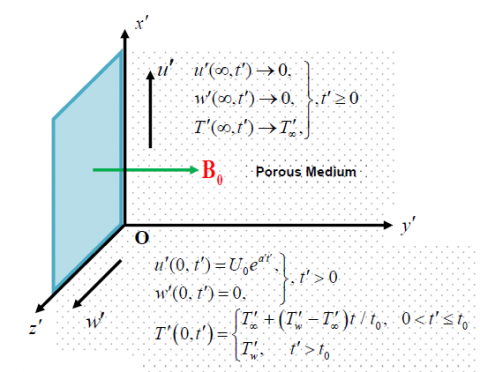Figure 1. Geometry of the problem

Since plate is of infinite extent in $x^{\prime}$  and $z^{\prime}$  directions and is electrically non-conducting, all physical quantities except pressure, depend on $y^{\prime}$ and $t^{\prime}$  only. It is assumed that the induced magnetic field produced by fluid motion is negligible in comparison to the applied one. So the magnetic field $\vec{B} \equiv\left(0, B_{0}, 0\right)$.  This assumption is justified because magnetic Reynolds number is very small for metallic liquids and partially ionized fluids which are commonly used in industrial processes. Also no external electric field is applied so the effects of polarization of fluid are negligible.

With these assumptions, the governing equations for unsteady hydromagnetic natural convection flow of an electrically conducting, viscous, incompressible and temperature dependent heat absorbing fluid in a uniform porous medium, under Boussinesq approximation, taking Hall effects into account, in non-dimensional form (Seth et al. ), are given by

$\frac{\partial F}{\partial t}=\frac{\partial^{2} F}{\partial y^{2}}-(1-i m) \frac{M F}{\left(1+m^{2}\right)}-\frac{F}{K_{1}}+G_{r} T$       (1)

$\frac{\partial T}{\partial t}=\frac{1}{P_{r}} \frac{\partial^{2} T}{\partial y^{2}}-\phi T$   (2)

Where F=u+iw.

The non-dimensional variables and parameters used, are defined as

$y=y^{\prime} / U_{0} t_{0}, u=u^{\prime} / U_{0}, w=w^{\prime} / U_{0}, t=t^{\prime} / t_{0}, a=a^{\prime} t_{0}$

$T=\left(T^{\prime}-T_{\infty}^{\prime}\right) /\left(T_{w}^{\prime}-T_{\infty}^{\prime}\right), G_{r}=g \beta^{\prime} v\left(T_{w}^{\prime}-T_{\infty}^{\prime}\right) / U_{0}^{3}$

$K_{1}=K^{\prime} U_{0}^{2} / v^{2}, M=\sigma B_{0}^{2} v / \rho U_{0}^{2}, P_{r}=v / \alpha^{\prime}$ and

$\phi=v Q_{0} / \rho c_{p} U_{0}^{2}$

Where $m, M, K_{1}, P_{r}, \phi$ and $G_{r}$,  are respectively, Hall current parameter, Magnetic parameter, permeability parameter, Prandtl number, heat absorption parameter and Grashof number.

Initial and boundary conditions, in non-dimensional form, are given by

$F=0, T=0 \quad$ for $y \geq 0$ and $t \leq 0$     (3a)

$F=e^{a t} \quad$ at $y=0$ for $t>0$   (3b)

$T=t \quad$ at $y=0$ for $0<t \leq 1$     (3c)

$T=1 \quad$ at $y=0$ for $t>1$     (3d)

$F \rightarrow 0, T \rightarrow 0 \quad$ as $y \rightarrow \infty$ for $t>0$    (3e)

It is obvious from equations (1) and (2) that energy equation (2) is uncoupled from the momentum equation (1). Therefore, we have to obtain first the solution for fluid temperature $T(y, t)$  by solving equation (2) and then the solution for fluid velocity $F(y, t)$  has to be obtained with the use of solution for $T(y, t)$ in equation (1).

Using Laplace transform technique, exact solution for fluid temperature $T(y, t)$  and fluid velocity $F(y, t)$  is obtained and is presented in the following form after simplification:

(i) For $P_{r} \neq 1$

$T(y, t)=P(y, t)-H(t-1) P(y, t-1)$    (4)

$F(y, t)=e^{a t}\left[f_{2}(y, 1, \lambda, a, t)-\frac{\alpha}{2 \beta^{2}}[G(y, t)\right. -H(t-1) G(y, t-1)]]$   (5)

(ii) For $P_{r}=1$

$T(y, t)=P_{1}(y, t)-H(t-1) P_{1}(y, t-1)$   (6)

$F(y, t)=e^{a t}\left[f_{1}(y, 1, \lambda, a, t)\right]-\gamma\left[G_{1}(y, t)-H(t-1) G_{1}(y, t-1)\right]$    (7)

Where $P(y, t)=f_{1}\left(y, t, P_{r}, \phi\right)$

$G(y, t)=\frac{1}{2}\left[e^{\beta t}\left\{f_{2}(y, 1, \lambda, \beta, t)-f_{2}\left(y, P_{r}, \phi, \beta, t\right)\right\}\right. \left.-\beta\left\{f_{3}(y, 1, \lambda, \beta, t)-f_{3}\left(y, P_{r}, \phi, \beta, t\right)\right\}\right]$

$P_{1}(y, t)=\left[f_{1}(y, t, 1, \phi)\right]$

$G_{1}(y, t)=1 / 2\left[f_{1}(y, t, 1, \lambda)-f_{1}(y, t, 1, \phi)\right]$

$\alpha=G_{r} /\left(1-P_{r}\right), \gamma=G_{r} /(\lambda-\phi), \lambda=M /(1+i m)+1 / K_{1}$

and $\beta=\left(\phi P_{r}-\lambda\right) /\left(1-P_{r}\right)$

Expressions for $f_{1}, f_{2}$ and $f_{3}$ are defined in Appendix-I.

2.1 Shear stress and rate of heat transfer at the plate

Expressions for the primary shear stress $\tau_{x}$  secondary shear stress $\tau_{z}$  and rate of heat transfer at the plate $N_{u}$  are obtained and are presented in the following form

$\tau=\frac{e^{a t}}{2}\left[f_{4}(\lambda, 1, a, t)\right]-\frac{\alpha}{2 \beta^{2}} \times\left[G_{2}(0, t)-H(t-1) G_{2}(0, t-1)\right]$  (8)

$N_{u}=P_{2}(0, t)-H(t-1) P_{2}(0, t-1)$   (9)

Where $\tau=\tau_{x}+i \tau_{z}$

$G_{2}(0, t)=e^{\beta t}\left[f_{4}(\lambda, 1, \beta, t)-f_{4}\left(\phi, \mathrm{P}_{r}, \beta, t\right)\right] -\beta\left[f_{5}(\lambda, 1, \beta, t)+\frac{2}{\sqrt{\pi t}}\left(t+\frac{1}{\beta}\right) e^{-\lambda t}+\right. \left.\frac{2}{\sqrt{\pi t}}\left(t+\frac{1}{\beta}\right) e^{-\phi t}+f_{5}\left(\phi, \mathrm{P}_{r}, \beta, t\right)\right]$

Expressions for $f_{4}$ and $f_{5}$ are defined in Appendix-I.

3. Results and Discussions

To study the nature of flow-field, the numerical values of primary velocity $\mathcal{U}$  and secondary velocity \mathcal{W}  in the boundary layer region, computed  from the analytical solution (5) are displayed graphically versus boundary layer coordinate $y$  for various values of plate acceleration parameter $a$ , Hall current parameter $m$ , heat absorption parameter $\phi$   and time $t$  in Figures 2 to 5 taking magnetic parameter $M=10$,  permeability parameter $K_{1}=0.4$ and Prandtl number $P_{r}=0.71$  (ionized air).

It is revealed from Figures 2 to 5 that, secondary fluid velocity $w$  attains a distinctive maximum value in the region near the plate and then decreases properly on increasing boundary layer coordinate $y$  to approach free stream value. It is noticed from Figure 2 that, $u$ and $w$  increase on increasing $a$ . This implies that plate acceleration parameter tends to accelerate fluid flow in both the primary and secondary flow directions. It is revealed from Figure 3 that $u$  and $W$  increase on increasing $m$ . This implies that Hall current tends to accelerate fluid flow in both the primary and secondary flow directions. This is due to the reason that Hall current induces secondary flow in the flow field. It is noticed from Figure 4 that $u$  and $w$  decrease on increasing $\phi$ . This implies that heat absorption tends to retard fluid flow in both the primary and secondary flow directions. It is evident from Figure 5 that $u$  and $w$  increase on increasing $t$ This implies that primary and secondary fluid velocities are getting accelerated with the progress of time.

The numerical values of fluid temperature $T$  computed from analytical solution (4), are displayed graphically versus boundary layer coordinate $y$  in Figures 6 and 7 for various values of heat absorption parameter $\phi$   and time $t$  It is noticed from Figures 6 and 7 that $T$ decreases on increasing $\phi$  whereas it increases on increasing $t$ . This implies that heat absorption tends to reduce fluid temperature. As time progresses, there is an enhancement in fluid temperature. It is observed from Figures 6 and 7 that fluid temperature $T$  attains its maximum value at the surface of the plate and it decreases properly on increasing boundary layer co-ordinate $y$  to approach free stream value.

The numerical values of shear stress $\tau_{x}$  and $\tau_{z}$  at the plate due to primary and secondary flows respectively, computed from analytical expression (8), are presented in the tabular form in the Tables 1 and 2 for various values of $a, m, \phi$ and $t$ taking $M=10, K_{1}=0.4$  and $P_{r}=0.71$  whereas those of rate of heat transfer $N_{u}$  at the plate, calculated from the analytical expression (9), are provided in Table 3 for different values of $\phi$ and $t$  taking P_{r}=0.71.  It is evident from Table 1 that primary shear stress $\tau_{x}$  at the plate decreases on increasing $m$  whereas it increases on increasing $a$ . Secondary shear stress $\tau_{z}$  at the plate increases on increasing either $m$  or $a$ . This implies that plate acceleration parameter tends to enhance primary as well as secondary shear stress at the plate. Hall current tends to reduce primary shear stress at the plate whereas it has a reverse effect on the secondary shear stress at the plate. It is found from Table 2 that $\tau_{x}$  increases on increasing either $\phi$ or $t$  whereas  \tau_{z}  decreases on increasing either $\phi$ or $t$ . This implies that primary shear stress at the plate is getting enhanced whereas secondary shear stress at the plate is getting reduced with an increase in heat absorption and with the progress of time. It is evident from Table 3 that rate of heat transfer $N_{u}$  at the plate increases on increasing either $\phi$ or $t$. This implies that heat absorption tends to enhance rate of heat transfer at the plate. As time progresses, rate of heat transfer at the plate is getting enhanced.

4. Conclusion

Noteworthy results are summarized below. Plate acceleration parameter and Hall current tend to accelerate fluid flow in both the primary and secondary flow directions. Heat absorption tends to decelerate fluid flow in both the primary and secondary flow directions. Primary and secondary fluid velocities are getting accelerated with the progress of time. Heat absorption tends to reduce the fluid temperature. Fluid temperature is enhanced with the progress of time. Plate acceleration parameter tends to enhance primary and secondary shear stress at the plate. Hall current tends to reduce the primary shear stress at the plate whereas these agencies have reverse effect on the secondary shear stress at the plate. Heat absorption tends to enhance primary shear stress at the plate whereas it has a reverse effect on the secondary shear stress at the plate. Primary shear stress at the plate is getting enhanced whereas secondary shear stress at the plate is getting reduced with the progress of time. Heat absorption tends to enhance rate of heat transfer at the plate. Rate of heat transfer at the plate is getting enhanced with progress of time.

## 2.png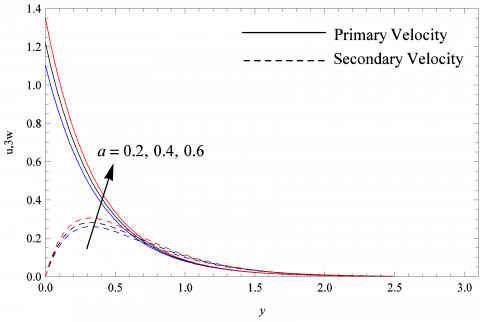Figure 2. Velocity profiles when m=0.5,$G_{r}=10, \phi=3$ and $t=0.5$

## 3.png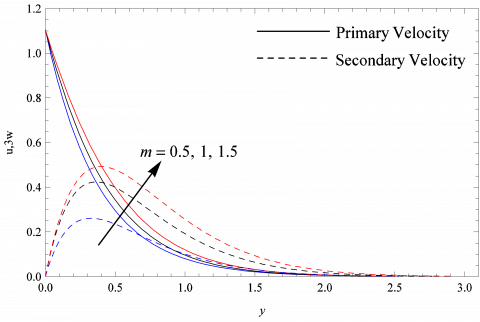Figure 3. Velocity profiles when $a=0.2$,$G_{r}=10, \phi=3$ and $t=0.5$

## 4.png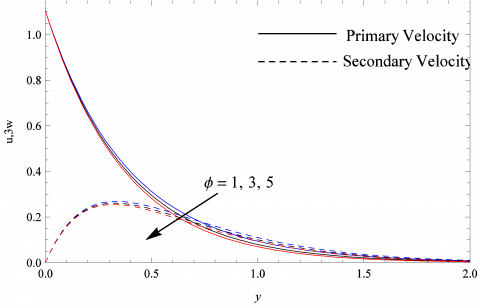Figure 4. Velocity profiles when $a=0.2$,$m=0.5, G_{r}=10,$ and $t=0.5$

## 5.png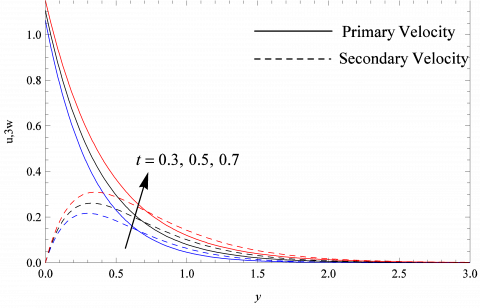Figure 5. Velocity profiles when $a=0.2$,$m=0.5, G_{r}=10$ and $\phi=3$

## 6.png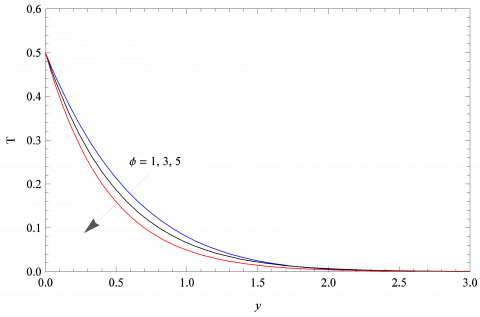Figure 6. Temperature profiles when $t=0.5$

## 7.png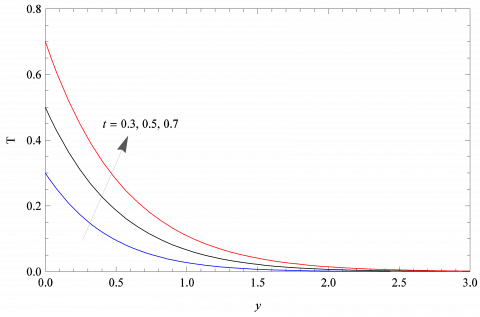Figure 7. Temperature profiles when $\phi=3$

Table 1. Shear stress at the plate when $\phi=3$  and $t=0.5$

 $m \downarrow a \rightarrow$ 0.2 0.8 1.4 $-\tau_{x}$ 0.5 6.3152 9.1449 13.0477 1.0 5.3550 7.8819 11.3826 1.5 4.5521 6.8443 10.0359 $\tau_{z}$ 0.5 1.499 1.91875 2.4735 1.0 2.2295 2.81451 3.5849 1.5 2.5084 3.10022 3.8763

Table 2. Shear stress at the plate when a=0.2 and m=0.5

 $\phi \downarrow t \rightarrow$ 0.3 0.5 0.7 $-\tau_{x}$ 1 6.1317 6.2304 6.6870 3 6.2948 6.3152 6.7591 5 6.3977 6.4526 6.8082 $\tau_{z}$ 1 1.5962 1.5716 1.5598 3 1.5469 1.539 1.5158 5 1.5119 1.5028 1.4817

Table 3. Rate of heat transfer at the plate when $P_{r}=0.71$

 $t \downarrow \phi \rightarrow$ 1 3 5 $-N_{u}$ 0.3 0.096 0.2821 0.4185 0.5 0.2258 0.5527 0.7688 0.7 0.3754 0.8312 1.1198
Nomenclature
 $a$ Plate acceleration parameter $B_{0}$ Uniform magnetic field $c_{p}$ Specific heat at constant pressure $g$ Acceleration due to gravity $G_{r}$ Thermal Grashof number $K^{\prime}$ Permeability of porous medium $K_{1}$ Permeability parameter $m$ Hall current parameter $M$ Magnetic parameter $P_{r}$ Prandtl number $Q_{0}$ Heat absorption coefficient $T^{\prime}$ Fluid temperature $t_{0}$ Characteristic time $u^{\prime}$ Fluid velocity in $x^{\prime}$  direction $w^{\prime}$ Fluid velocity in $z^{\prime}$  direction Greek letters $\alpha^{\prime}$ Thermal diffusivity $\beta^{\prime}$ Coefficient of thermal expansion $\sigma$ Electrical conductivity $\rho$ Fluid density $v$ Kinematic coefficient of viscosity $\omega_{e}$ Cyclotron frequency $\tau_{e}$ Electron collision time $\phi$ Heat absorption parameter
Appendix-1

$f_{1}\left(\mathrm{c}_{1}, \mathrm{c}_{2}, \mathrm{c}_{3}, \mathrm{c}_{4}\right)=\left(\frac{1}{2}\right)\left[\left(c_{2}+\frac{c_{1}}{2} \sqrt{\frac{c_{3}}{c_{4}}}\right) e^{c_{1} \sqrt{a_{3} c_{4}}} \operatorname{erfc}\left(\frac{c_{1}}{2} \sqrt{\frac{c_{3}}{c_{2}}}\right.\right. +\sqrt{c_{4} c_{2}})+\left(c_{2}-\frac{c_{1}}{2} \sqrt{\frac{c_{3}}{c_{4}}}\right) e^{-c_{1} \sqrt{c_{3} c_{4}}} \times \operatorname{erfc}\left(\frac{c_{1}}{2} \sqrt{\frac{c_{3}}{c_{2}}}-\sqrt{c_{4} c_{2}}\right)$

$f_{2}\left(\mathrm{c}_{1}, \mathrm{c}_{2}, \mathrm{c}_{3}, \mathrm{c}_{4}, \mathrm{c}_{5}\right)=\mathrm{e}^{c_{1} \sqrt{c_{2}\left(\mathrm{c}_{3}+\mathrm{c}_{4}\right)}} \operatorname{erf}\left(\frac{c_{1}}{2} \sqrt{\frac{c_{2}}{c_{5}}}+\right. \sqrt{\left(c_{3}+c_{4}\right) c_{5}})-e^{-c_{1} \sqrt{c_{2}\left(c_{3}+c_{4}\right)}} \operatorname{erfc}\left(\frac{c_{1}}{2} \sqrt{\frac{c_{2}}{c_{5}}}-\sqrt{\left(\mathrm{c}_{3}+\mathrm{c}_{4}\right) \mathrm{c}_{5}}\right)$

$f_{3}\left(\mathrm{c}_{1}, \mathrm{c}_{2}, \mathrm{c}_{3}, \mathrm{c}_{4}, \mathrm{c}_{5}\right)=\left(c_{5}+\frac{1}{c_{4}}+\frac{c_{1}}{2} \sqrt{\frac{c_{2}}{c_{3}}}\right) e^{c_{1} \sqrt{c_{2} c_{3}}} \operatorname{erfc}\left(\frac{c_{1}}{2} \sqrt{\frac{c_{2}}{c_{5}}}+\sqrt{c_{3} c_{5}}\right) \times +\left(c_{5}+\frac{1}{c_{4}}-\frac{c_{1}}{2} \sqrt{\frac{c_{2}}{c_{3}}}\right) e^{-c_{1} \sqrt{c_{2} c_{3}}} \times \operatorname{erfc}\left(\frac{c_{1}}{2} \sqrt{\frac{c_{2}}{c_{5}}}-\sqrt{c_{3} c_{5}}\right)$

$f_{4}\left(c_{1}, c_{2}, c_{3}, c_{4}\right)=\frac{2 e^{-\left(c_{1}+c_{3}\right) c_{4}}}{\sqrt{\pi t}}+2 \sqrt{\left(c_{1}+c_{3}\right) c_{2}} \times \{\operatorname{erfc}(\sqrt{\left(c_{1}+c_{3}\right) c_{4}})-1\}$

$f_{5}\left(c_{1}, c_{2}, c_{3}, c_{4}\right)=2\left\{\sqrt{c_{1} c_{2}}\left(c_{4}+\frac{1}{c_{3}}\right)+\frac{1}{2} \sqrt{\frac{c_{2}}{c_{1}}} \times\right. \{\operatorname{erfc}(\sqrt{c_{1} c_{4}})\}-1\}$

References

1. M. Faraji and H. E. L.  Qarnia, Numerical Optimization of a Thermal Performance of a Phase Change Material Based Heat Sink, Int. J. Heat & Tech., vol. 26, pp. 17–24, 2008.

2. E. M. Sparrow and R. D. Cess, Temperature-dependent Heat Sources or Sinks in a Stagnation Point Flow, Appl. Sci. Res., vol. 10, pp. 185-197, 1961. DOI: 10.1007/BF00411912.

3. D. Moalem, Steady State Heat Transfer within Porous Medium with Temperature Dependent Heat Generation, Int. J. Heat Mass Tran., vol. 19, pp. 529-537, 1976. DOI: 10.1016/0017-9310(76)90166-6.

4. M. H. Kamel, Unsteady MHD Convection  through Porous Medium with Combined Heat and Mass Transfer with Heat Source/Sink, Energ. Convers. Manage., vol. 42, pp. 393-405, 2001. DOI: 10.1016/S0196-8904(00)00067- 4.

5. A. J. Chamkha, Unsteady MHD Convective Heat and Mass Transfer Past a Semi-Infinite Vertical Permeable Moving Plate with Heat Absorption, Int. J. Eng. Sci., vol. 42, pp. 217-230, 2004. DOI: 10.1016/S0020- 7225(03)00285-4.

6. M. Ferdows, K. Kaino and J. C. Crepeau, Natural Convection of a Magnetohydrodynamic Flow Past a Semiinfinite Vertical Porous Plate in a Porous Medium with Internal Heat Generation, Int. J. Heat & Tech., vol. 25, pp. 91-94, 2007.

7. M. Ferdows, J. C. Crepeau and A. Postelnicu, Natural Convection Flow with Wall Temperature Considering Internal Heat Generation, Int. J. Heat & Tech, Vol. 27, pp. 107-110, 2009.

8. P. Chandran, N. C. Sacheti and A. K. Singh, Natural Convection near a Vertical Plate with Ramped Wall Temperature, Heat Mass Transfer, vol. 41, pp. 459-464, 2005. DOI: 10.1007/s00231-004-0568-7.

9. G. S. Seth and Md. S. Ansari, MHD Natural Convection Flow Past an Impulsively Moving Vertical Plate with Ramped Wall Temperature in the Presence of Thermal Diffusion with Heat Absorption, Int. J. Appl. Mech. Eng. vol. 15, pp. 199-215, 2010.

10. Samiulhaq, I. Khan, F. Ali and S. Shafie, MHD Free Convection Flow in a Porous Medium with Thermal Diffusion and Ramped Wall Temperature, J. Phys. Soc. Jpn., vol. 81, 2012. DOI: 10.1143/JPSJ.81.044401.

11. K. Das, Magnetohydrodynamics Free Convection Flow of a Radiating and Chemically Reacting Fluid Past an Impulsively Moving Plate with Ramped Wall Temperature, J. Appl. Mech., vol. 79, 2012. DOI: 10.1115/1.4006462.

12. R. Nandkeolyar, M. Das and P. Sibanda, Exact Solutions of Unsteady MHD Free Convection in a Heat Absorbing Fluid Flow Past a Flat Plate with Ramped Wall Temperature, Bound. Value. Probl., vol. 1, pp. 247, 2013. DOI: 10.1186/1687-2770-2013-247.

13. P. K. Kundu, K., Das and N. Acharya, Flow Features of a Conducting Fluid Near an Accelerated Vertical Plate in Porous Medium with Ramped Wall Temperature, J. Mech. vol. 30, pp. 277-288, 2014. DOI: 10.1017/jmech.2014.14.

14. G. W. Sutton and A. Sherman, Engineering Magnetohydrodynamics, McGraw-Hill, New York, 1965.

15. G. S. Seth, S. Sarkar and G. K. Mahato, Effects of Hall Current on Hydromaggnetic Free Convection Flow with Heat and Mass Transfer of a Heat Absorbing Fluid Past an Impulsively Moving Vertical Plate with Ramped Temperature, Int. J. Heat & Tech. vol. 31, pp. 85-96, 2013.